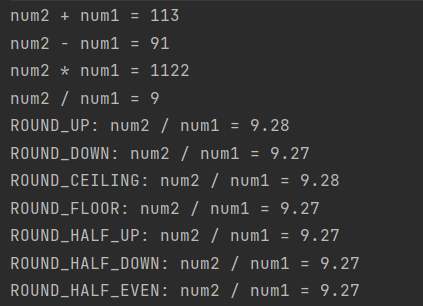# Usage of Bigdecimal

As a `Java` developer in the daily work, many times we will encounter some need to calculate the data scenario, usually for the scenario does not need to calculate the precision we can use `Integer`, `Float` or `Double` to calculate, although will lose precision but occasionally can be used, if we need to calculate the results accurately, we will use the class BigDecimal provided in the java.math package to implement the corresponding function.

`BigDecimal` is a tool for accurate data calculation, since it is a data calculation, it will definitely provide the corresponding methods of addition, subtraction, multiplication and division for us to use, as follows.

1. `add(BigDecimal)` : Add the values in the `BigDecimal` object and return the `BigDecimal` object
2. `subtract(BigDecimal)` : The values in `BigDecimal` object are subtracted, and `BigDecimal` object is returned.
3. `multiply(BigDecimal)` : Multiply the values in the `BigDecimal` object and return the `BigDecimal` object
4. `divide(BigDecimal)` : Divide the values in the `BigDecimal` object, and return the `BigDecimal` object.

When we need to use the corresponding method, we need to create `BigDecimal` object first, and then we can use it, and the corresponding constructor methods are

1. `BigDecimal(int)` : Create an object with the integer value specified by the parameter
2. `BigDecimal(double)` : Creates an object with the double precision value specified by the parameter
3. `BigDecimal(long)` : Creates an object with the long integer value specified by the parameter
4. `BigDecimal(String)` : Creates an object with the value specified by the parameter as a string.

After the `BigDecimal` object is created by the constructor method, the corresponding method is called and another `BigDecimal` parameter is passed to achieve the corresponding addition, subtraction, multiplication and division method. The following example is shown.

``````package org.test;

import java.math.BigDecimal;

public class TestClass {

public static void main(String[] args) {
BigDecimal num1 = new BigDecimal("11");
BigDecimal num2 = new BigDecimal("102");
BigDecimal result2 = num2.subtract(num1);
BigDecimal result3 = num2.multiply(num1);
System.out.println("num2 + num1 = " + result1);
System.out.println("num2 - num1 = " + result2);
System.out.println("num2 * num1 = " + result3);
System.out.println("num2 / num1 = " + (102 / 11));
System.out.println("ROUND_UP: num2 / num1 = " + num2.divide(num1, 2, BigDecimal.ROUND_UP));
System.out.println("ROUND_DOWN: num2 / num1 = " + num2.divide(num1, 2, BigDecimal.ROUND_DOWN));
System.out.println("ROUND_CEILING: num2 / num1 = " + num2.divide(num1, 2, BigDecimal.ROUND_CEILING));
System.out.println("ROUND_FLOOR: num2 / num1 = " + num2.divide(num1, 2, BigDecimal.ROUND_FLOOR));
System.out.println("ROUND_HALF_UP: num2 / num1 = " + num2.divide(num1, 2, BigDecimal.ROUND_HALF_UP));
System.out.println("ROUND_HALF_DOWN: num2 / num1 = " + num2.divide(num1, 2, BigDecimal.ROUND_HALF_DOWN));
System.out.println("ROUND_HALF_EVEN: num2 / num1 = " + num2.divide(num1, 2, BigDecimal.ROUND_HALF_EVEN));
}
}
``````

The result of the run is shown below.From the above figure, we can see that BigDecimal is used in a specific way by calling the corresponding method and passing in the corresponding parameters. However, when dividing, we can see that the divide method also provides the parameter to set the exact number of digits, and it is possible to set the specific rounding method. There are several integer methods.

``````// absolute value upward integer, away from the coordinate draw 0 integer
public final static int ROUND_UP = 0;
// absolute value downward rounding, towards the coordinates of the city 0 rounding
public final static int ROUND_DOWN = 1;
//value direction upward rounding, rounding to positive infinity
public final static int ROUND_CEILING = 2;
//Value direction downward rounding, rounding to negative infinity
public final static int ROUND_FLOOR = 3;
// >= 0.5 absolute value direction up to integer, < 0.5 absolute value direction down to integer
public final static int ROUND_HALF_UP = 4;
// <= 0.5 absolute value direction downward rounding, > 0.5 absolute value direction upward rounding
public final static int ROUND_HALF_DOWN = 5;
// rounding mode to the "nearest neighbor" rounding, unless the two neighbors are equally spaced, in which case, rounding to the even number of neighbors. If the number to the left of the discarded fraction is odd, the // behavior is the same as RoundingMode.HALF_UP; if it is even, it behaves as RoundingMode.HALF_DOWN
public final static int ROUND_HALF_EVEN = 6;
public final static int ROUND_UNNECESSARY = 7;
``````

In addition to the methods of addition, subtraction, multiplication and division, BigDecimal also provides the method compareTo() for comparing two BigDecals, the usage is as follows

``````BigDecimal num1 = new BigDecimal("101");
BigDecimal num2 = new BigDecimal("102");
int i = num2.compareTo(num1);
System.out.println(i);
// Run result: 1
``````

Because num2 is larger than num1, the return value is 1; when num2 is equal to num1, it returns 0; when num2 is smaller than num1, it returns -1.

The summary format is as follows

``````int a = bigdemical.compareTo(bigdemical2);
//a = -1, means bigdemical is smaller than bigdemical2.
//a = 0,means bigdemical is equal to bigdemical2.
//a = 1,means bigdemical is greater than bigdemical2.
``````

`if (num2.compareTo(num1) > 0)` judgment is a common operation.

Also BigDecimal provides methods to convert directly to int, long, float, double numeric values as follows, and it is used relatively rarely in general.

``````int i1 = num1.intValue();
long l = num1.longValue();
float v = num1.floatValue();
double v1 = num1.doubleValue();
short i2 = num1.shortValue();
byte b = num1.byteValue();
``````

Use `BigDecimal` when you need accurate decimal calculation in your daily work, the performance of `BigDecimal` is relatively worse than `double` and `float`, so use it only when you need it. `BigDecimal` creates a new object every time we add, subtract, multiply and divide, and we need to save it when we need to use it later, so usually we try to use the constructor of String type.